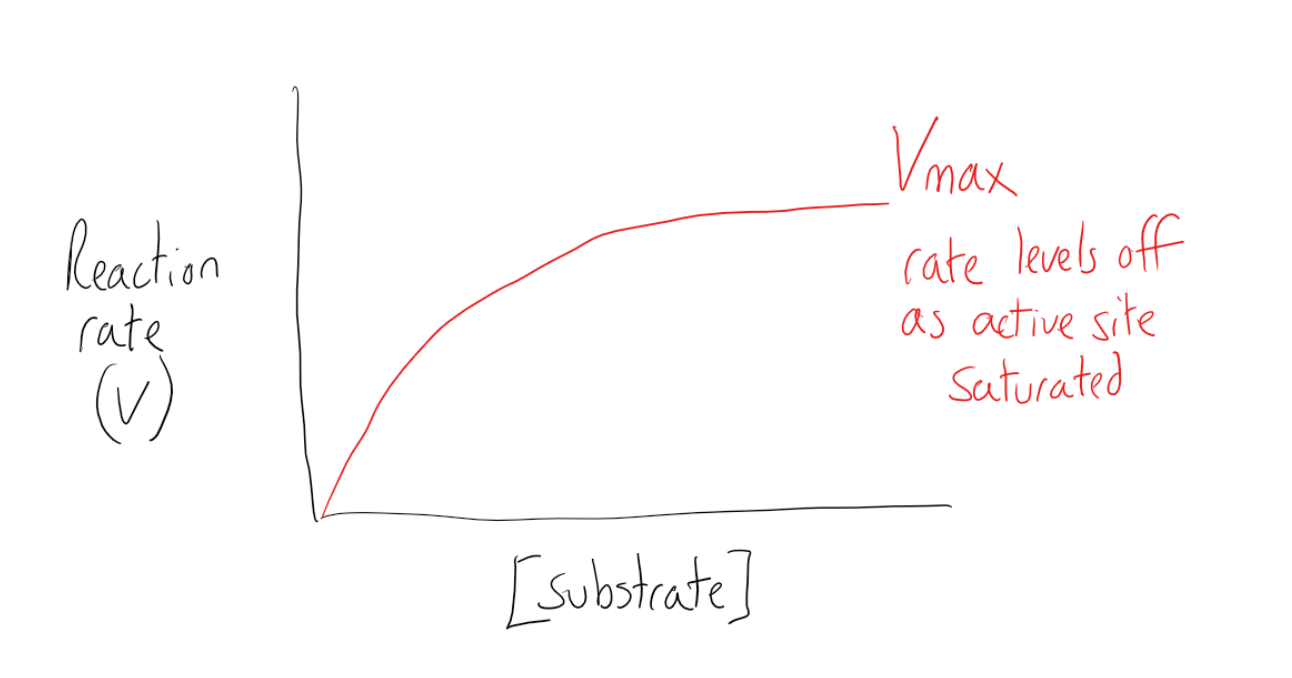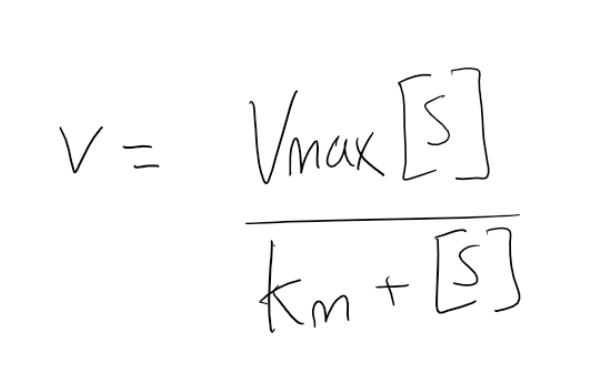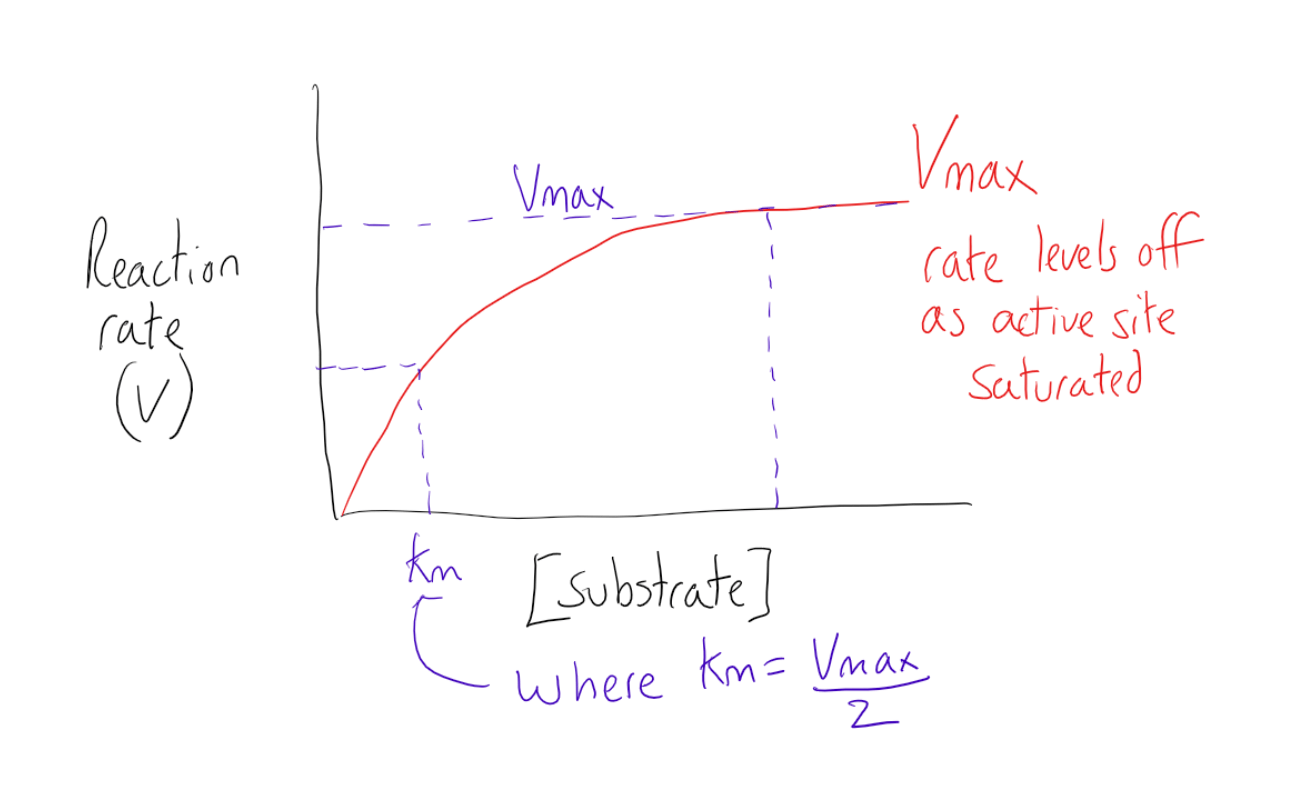### Kinetics

Topic: Control Of Enzyme Activity

Enzyme kinetics is the study of the rates of chemical reactions that are catalyzed by enzymes.

A substance that helps a chemical reaction to occur is a catalyst, and the special molecules that catalyze biochemical reactions are called enzymes. Enzymes do this by binding to the reactant molecules and holding them in such a way as to make the chemical bond-breaking and bond-forming processes take place more readily. This occurs when an enzyme-substrate complex forms in the equation:

E+S -> ES -> E + P
where S is the substrate, E is the enzyme and P is the product
**Remember, enzymes do not change the ∆G of a reaction**

When measuring the change of a reaction, we can deduce a rate equation. If we use the general equation A -> B where A is a substrate and B is a product, the rate equation is given as rate = k(A). But as an enzyme will take part in this reaction, we end up with a two-step reaction E+S -> ES -> E + P becomes E + S -> ES and ES -> E + P. This results in two rate equations, making this reaction a multi-step reaction:

Rate 1 = K1 (E)(S)
Rate 2 = K2 (ES)

By considering these two equations, there are two ways that we can speed up the rate of reaction:

1) By increasing the substrate concentration
2) Increasing the enzyme concentration

We must assume that the concentration of the enzymes is constant and the maximum rate (Vmax) occurs when there is a high substrate concentration and all active sites of the enzyme are saturated. Increasing the substrate concentration beyond this point will have no effect on the reaction rate as the enzyme active sites are saturated.This kinetic relationship makes two key assumptions, however:

1. That the solutions behave ideally and the enzymes bond to the substrate to form the complex and then the complex forms products in 2 steps. There is no inhibition or allosteric activation.
2. The conditions around the reaction such as temperature and pH are constant and the concentration of enzyme is kept the same.

Michaelis Menten Kinetics

Michaelis Menten is another kinetic model for examining the reaction of substrates and enzymes. The equation relates the reaction rate V and formation of product (P) to the concentration of the substrate (S). It also makes use of the Vmax (the maximum rate achieved in the reaction when the enzyme is saturated) and the Michaelis constant Km (which is numerically equal to the substrate concentration at which the reaction rate is half of Vmax). This equation can be used to work out the rate of the formation of products for reactions that involve single substrates.The Michaelis Menten equation relies on the calculation of Vmax and Km. These can be determined by running a series of experiments with varying concentrations and measuring the initial reaction rate. By plotting the rate against concentration, the Vmax and Km can be obtained.Cooperativity

When enzymes contain more than one active site, the binding of a substrate molecule to the first site may influence substrate binding to a second site. As each subsequent bonding can impact the following site, this phenomenon is called cooperativity.

There are a few types of cooperativity:

Positive cooperative binding increases the affinity of an enzyme for a subsequent substrate molecule.
Negative cooperative binding decreases the enzymes affinity for subsequent binding of a substrate
Non-cooperative binding occurs when substrate binding does not affect affinity for subsequent substrates.Practice Questions

Biochemistry of a newly discovered (pretend) neurotransmitter

Mechanisms of antibody variability during B-cell development

Sarin gas and its potent effects on the body

The genetic basis of Phenylketonuria

Glucogenic and ketogenic amino acids

MCAT Official Prep (AAMC)

Section Bank C/P Section Question 98

Online Flashcards Biochemistry Question 4

Key Points

• Enzyme kinetics is the study of the rates of chemical reactions that are catalyzed by enzymes.

• Enzyme kinetics focuses on two key stages, the binding of the enzyme to the substrate to form the ES complex and the reaction of the ES complex to form product creating a two-step reaction.

• There are two ways to accelerate the rate of reaction: increasing the substrate concentration  OR increasing the enzyme concentration.

• Vmax is the maximum rate achieved where the enzyme active site is saturated.

• The Michaelis Menten equation can be used to work out the rate of a reaction by relating the Vmax and Michaelis constant (Km) to the substrate concentration.

• Cooperativity relates to when enzymes contain more than one active site and the binding of a substrate molecule to the one site may influence substrate binding to a subsequent site.

Key Terms

Active site: The active site is the part of an enzyme to which substrates bind and where a reaction is catalyzed.

Enzyme substrate complex: A temporary molecule formed when an enzyme binds to a substrate

Billing Information